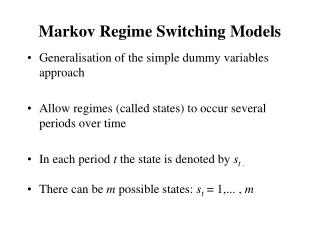DownloadDownload PresentationMarkov Regime Switching Models

# Markov Regime Switching Models

Download Presentation## Markov Regime Switching Models

- - - - - - - - - - - - - - - - - - - - - - - - - - - E N D - - - - - - - - - - - - - - - - - - - - - - - - - - -
##### Presentation Transcript

1. Markov Regime Switching Models • Generalisation of the simple dummy variables approach • Allow regimes (called states) to occur several periods over time • In each period t the state is denoted by st . • There can be m possible states: st = 1,... , m

2. Markov Regime Switching Models Example: Dating Business Cycles • Let yt denote the GDP growth rate - Simple model with m= 2 (only 2 regimes) yt = 1 + et when st = 1 yt = 2 + et when st = 2 e t i.i.d. N(0,2) - Notation using dummy variables: yt = 1 D1t + 2 (1-D1t) + et where D1t = 1 when st = 1, = 0 when st = 2

3. Markov Regime Switching Models • How does st evolve over time? • Model: Markov switching : P[sts1, s2, ..., st-1] = P[stst-1] • Probability of moving from state i to state j: pij = P[st =jst-1=i]

4. Markov Regime Switching Models • Example with only 2 possible states: m=2 • Switching (or conditional) probabilities: Prob[st = 1  st-1 = 1] = p11 Prob[st = 2  st-1 = 1] = 1 - p11 Prob[st = 2  st-1 = 2] = p22 Prob[st = 1  st-1 = 2] = 1 – p22

5. Markov Regime Switching Models • Unconditional probabilities: Prob[st = 1] = (1-p22 )/(2 - p11 - p22 ) Prob[st = 2] = 1 - Prob[st = 1] Examples: If p11 =0.9 and p22 =0.7 then Prob[st = 1] =0.75 If p11 =0.9 and p22 =0.9 then Prob[st = 1] =0.5

6. Markov Regime Switching Models Estimation: • Maximize Log-Likelihood • Hamilton filter - Filtered probabilities:  1, t = Prob[st = 1 | y1, y2, …, yt ]  2 ,t = Prob[st = 2 | y1, y2, …, yt ] = 1-  2 ,t for t=1, …, T

7. Markov Regime Switching Models GDP growth rate

8. Markov Regime Switching Models Estimated model: yt = 0.96 + et when st = 1 yt = -0.52 + et when st = 2 e t i.i.d. N(0,0.782) p11 = 0.95 p22 = 0.79

9. Markov Regime Switching Models  2 ,t : filtered probability of being in state 2